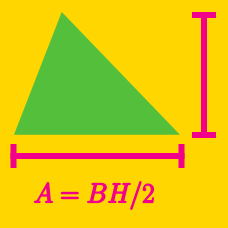Geometry

# Area of Triangles - Shoelace Formula

Let $A=(5,2)$ and $B=(6,-5)$ be two vertices of triangle $ABC.$ If the area of the triangle is $9,$ which of the following are possible coordinates for the third vertex?

Consider a square $ABCD$ with side length $19.$ If we let $M$ and $N$ be the midpoints of $\overline{BC}$ and $\overline{CD},$ respectively. What is the area of $\triangle AMN?$

Statement $1$: If the area of the triangle bounded by the lines $y=x, x+y=8$ and the line through $P=(h,k)$ parallel to the $x$-axis is $4h^2,$ then $P$ lies on either of the two lines represented by $4x^2+8y-y^2-16=0.$

Statement $2$: The area of the triangle bounded by the lines $y=x, x+y=8$ and the $x$-axis is equal to half of the area of the triangle bounded by the line $x+y=8$ and the two coordinate axes.

Which of the following is true of the above two statements?

Triangle $ABC$ has vertices $A=(5, 6)$ and $B=(6, 3).$ If its area is $22$ and vertex $C$ lies on the line $y=x+1$ in the first quadrant, what are the coordinates of $C?$

Given three points $\begin{array}{c}&A=(-1,0), &B=(0,12), &C=(k, k),\end{array}$ where $k\ge 2,$ what is the value of $k$ that minimizes the area of $\triangle ABC?$

×